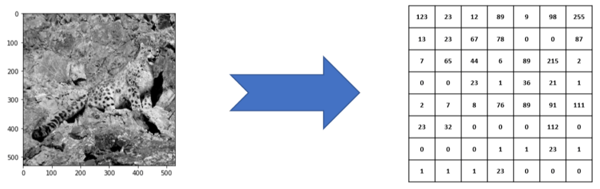Related Tags

python
communitycreator

# How to use scikit image thresholding methods in Python

Utkal Sinha

Images are just collections of numbers, also known as pixels. These numbers range from 0 to 255, where 0 represents a dark pixel and 255 represents a very bright pixel.

These numbers are represented in the form of a matrix or table, where an intersection of rows and columns denote a unique pixel in the image. This is done for a grey image below:Leopard Grey Image to pixels values representation

Color images are a superimposition of 3 layers, matrices, or tables that represent a red channel matrix, a green channel matrix, and a blue channel matrix. These 3 channels are commonly referred to as RGB coding of an image. This is shown below:Leopard Color Image to RGB layers pixels values representation

However, RGB is not the only color image encoding mechanism. There are other image color spaces such as YCbCr and CMYK. In this post, the RGB color space would be used.

## Thresholding

Thresholding is a simple image preprocessing or filtering method that replaces: each pixel value in the image matrix with:

• 0 (representing dark) if the existing pixel is less than the constant value K
• 255 (representing bright) if the existing pixel value is more than the constant value K

This K constant is known as the threshold value in the thresholding operation.

Since the thresholded image only contains two pixel values (0 and 255), the result of a thresholding operation is a binary image. Below is the thresholded image of the leopard image from earlier:Leopard binary image obtained using threshold_otsu from skimage.filters package

Below is the code snippet that will allow us to obtain the above binary image:

# Import the skimage threshold_otsu package
from skimage.filters import threshold_otsu

# get the global optimal threshold for the leopard image
globalthreshold = threshold_otsu(leopardGrayImg)

# apply the threshold to the gray image to obtain a binary image
leopardBinaryImg =leopardGrayImg > globalthreshold

# finally show the binary image
imshow(leopardBinaryImg)

The complete jupyter notebook is available in the following repo:

RELATED TAGS

python
communitycreator

CONTRIBUTOR

Utkal Sinha
RELATED COURSES

View all Courses

Keep Exploring

Learn in-demand tech skills in half the time